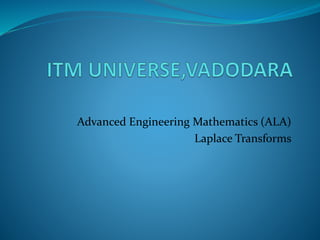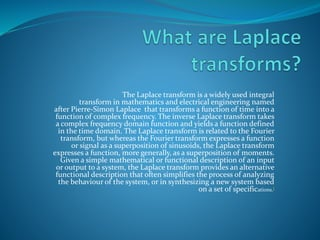Successfully reported this slideshow.

# Laplace transforms

6

Share×
1 of 16
1 of 16

# Laplace transforms

6

Share

## More Related Content

### Related Books

Free with a 14 day trial from Scribd

See all

### Related Audiobooks

Free with a 14 day trial from Scribd

See all

### Laplace transforms

1. 1. Advanced Engineering Mathematics (ALA) Laplace Transforms
2. 2. LAPLACE TRANSFORMS
3. 3. The Laplace transform is a widely used integral transform in mathematics and electrical engineering named after Pierre-Simon Laplace that transforms a function of time into a function of complex frequency. The inverse Laplace transform takes a complex frequency domain function and yields a function defined in the time domain. The Laplace transform is related to the Fourier transform, but whereas the Fourier transform expresses a function or signal as a superposition of sinusoids, the Laplace transform expresses a function, more generally, as a superposition of moments. Given a simple mathematical or functional description of an input or output to a system, the Laplace transform provides an alternative functional description that often simplifies the process of analyzing the behaviour of the system, or in synthesizing a new system based on a set of specifications.[
4. 4. The Laplace transform is a frequency domain approach for continuous time signals irrespective of whether the system is stable or unstable. The Laplace transform of a function(t), defined for all real numbers t ≥ 0, is the function F(s), which is a transform defined by:
5. 5. Multiplication by ‘t’
6. 6. Division by ‘t’
7. 7. APPLICATIONS OF LAPLACE TRANSFORMS
8. 8. Application in Electric Circuit Theory The Laplace transform can be applied to solve the switching transient phenomenon in the series or parallel RL,RC or RLC circuits . A simple example of showing this application follows next. Let us consider a series RLC circuit as shown in Fig 1. to which a d.c. voltage Vo is suddenly applied Now, applying Kirchhoff’s Voltage Law (KVL) to the circuit, we have, Ri + Ldi/dt + 1/C R idt = Vo (3) Differentiating both sides, Ld2 i/di2 + 1/Ci + Rdi/dt = 0; or, d2 i/dt2 + (R/L)di/dt + (1/LC)i = 0 (4) Now, applying laplace transform to this equation, let us assume that the solution of this equation is i(t) = Kest where K and s are constants which may be real, imaginary or complex. Now, from eqn (4), LKs2 e st + RKest + 1/CKest = 0 which on simplification gives, or, s2 + (R/L)s + 1/LC = 0
9. 9. Application in Power Systems Load Frequency control Power systems are comprised of generation, transmission and distribution systems. A generating system consists of a turbogenerator set in which a turbine drives the electrical generator and the generator serves the loads through transmission and distribution lines. It is required that the system voltage and frequency has to be maintained at some pre-specified standards eg. frequency have to be maintained at 50 or 60 Hz and voltage magnitude should be 0.95-1.05 per unit. In an interconnected power system, Load Frequency Control (LFC) and Automatic Voltage Regulator (AVR) equipment are installed for each generator. The controllers are set for a particular operating condition and take care of small changes in load demand to maintain the frequency and voltage within specified limits. Changes in real power is dependent on the rotor angle, δ and thus system frequency and the reactive power is dependent on the voltage magnitude that is, the generator excitation. In order to design the control system, the initial step is the modeling of generator, load, prime mover (turbine) and governer .
10. 10. Other applications: 1) Application of Laplace transforms for the solution of transient mass- and heat-transfer problems in flow systems 2)It is used in circuit analysis (in electrical engineering)
11. 11. THANKYOU